# Fun with shear operations and SVD – I – shear matrices and examples created with Blender

This post requires Javascript to display formulas!

In Machine Learning samples of data vectors often undergo linear transformations. Typical examples are the adaption of vector component values due to the choice of a different shifted and/or rotated coordinate system. Another example is the scaling of vectors. As we have seen in an investigation of Autoencoders they produce multivariate normal distributions which actually are the result of linear transformations of elementary Gaussian distributions. And let us not forget that the transport between layers of neural networks includes linear transformations.

In most cases we can interpret a linear transformations in the ℝn in a geometrical way. Linear transformations are members of a sub-class of affine transformations. Affine transformations in turn can be regarded as a combination of

• a translation,
• a scaling in either coordinate direction,
• a reflection = mirroring operation,
• a rotation
• and/or a shear operation.

Translations are trivial. We also understand the effects of rotation and scaling transformations intuitively. A mirror operation can be understand as a kind of inverted scaling with factors -1.

Scaling operations with different scaling factors along different coordinate directions destroy certain rotational symmetries of a 3-dimensional or n-dimensional body. But scaling transformations do not always eliminate symmetries with respect to mirroring planes: If and when we first choose an Euclidean coordinate system whose axes are aligned with symmetry axes of a body with plane symmetries, we are able to keep up at least some of the planar symmetries of the body during scaling operation.

Something that is a bit more difficult to grasp is a shear operation. The reason is that a shear operation destroys one or multiple plane symmetries of an impacted figure – whatever coordinate system we choose. And a shear operation cannot be reduced to a pure scaling operation in some cleverly chosen coordinate system. Neither can it be reduced to a rotation.

This alone is a good reason to have a closer look at this special kind of affine transformation. Another reason is to study what impact a shear operations has on the construction or transformation of a multivariate normal distribution – a topic which is at the center of another post series in this blog.

In this post I first want to discuss what a matrix that controls a shear operation looks like. We discuss some of its mathematical properties. Afterward I want to demonstrate what effects shearing has on two simple objects – a cube and a sphere. These examples will be done with Blender and the available mouse driven shear operations there.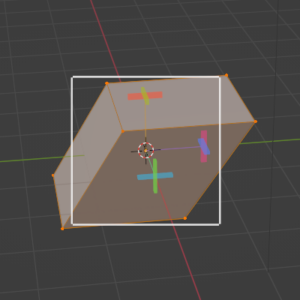Blender performs the required math in the background – and the examples below only provide visual impressions.

To get a better understanding of the nature of shear operation we, in the second post of this series, switch to Python and apply shear operations via explicit matrix operations onto vectors. We start with 2D-figures. Afterward we turn to 3D-cuboids and find that we produce spats. Another important example (not only with respect to multivariate normal distributions) is then given by the shearing of 3D-spheres: We will find that such an operation always produce ellipsoids. We will check this mathematically.

In a fourth post I want to discuss an important result from linear algebra, namely the so called SVD-decomposition of a linear transformation and its meaning in our context of shear operations. SVD tells us that we can always replace a shear operation by a sequence of simpler operations. We will test this statement for our 2D- and 3D-objects objects. We then also find that the creation of an ellipsoid at any orientation can be done by applying just one shear operation to a unit sphere. Alternatively, we can perform a scaling of a sphere and rotate it once. This will help us to better understand the creation of multivariate normal distributions in a parallel post series.

# Shear operations break symmetries of the affected figures

A shear operation Msh introduces an asymmetry into originally symmetric figures. It does so by coupling vector components in a specific way. For reasons of simplicity we reduce our argumentation to the ℝn. We describe objects in this space with the help of position vectors to points inside and on the surface of these objects. We specify the components of position vectors with respect to an Euclidean coordinate system [ECS].

To make things easier we assume that the origin of our ECS coincides with the symmetry center of the body we apply the shear operation to. If the body has plane and point symmetries then the origin is placed at the cutting lines or the cutting point of such planes; i.e., the ECS origin coincides with the symmetry-center of the body and coordinate planes will coincide with some of the symmetry planes. For the cases we look at – spheres, cubes, coboids – the ECS origin thus gets identical with the body’s volume center.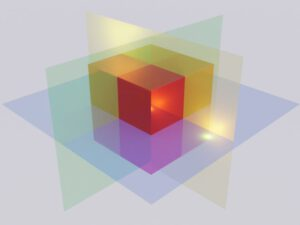A shear transformation applied to a vector couples at least two of the vector’s components such that a growing value for the coordinate value in the direction of a chosen coordinate axis (e.g. vz) impacts the coordinate value in another coordinate direction (e.g. vx) in a linear way.

Here is an example for the x- and z-components of a 3-dimensional vector (vx, vy, vz)T:

\begin{align} v_x^{sh} \, &= \, v_x \, + \, \alpha_{xz} * v_z \\ v_y^{sh} \,&=\, v_y \\ v_z^{sh}\,&=\, v_z \end{align}
$\pmb{\operatorname{M}}_{sh}: \quad \pmb{v} \,=\, \begin{pmatrix} v_x \\ v_y \\ v_z \end{pmatrix} \:\Rightarrow \: \pmb{v}_{sh} \,=\, \begin{pmatrix} v_x^{sh} \, = \, v_x \, + \, \alpha_{xz} * v_z \\ v_y^{sh} \,=\, v_y \\ v_z^{sh}\,=\, v_z \end{pmatrix},$

With α being some constant. This operation breaks an original plane symmetry of a body with respect to the x-z-plane.

## A shear operation preserves the orientation of tangent planes at some surface points

But note also, that a shear operation has another unique property that keeps up a geometrical property of the affected body:

A shear operation preserves the orientation of tangent planes to the body’s surface at some particular points. The most important of these points define extrema on the surface with respect to the component whose value is linearly added in the shearing transformation. The tangent planes there do not only keep up their orientation, but also their position.

In the case defined above we would look a extrema with respect to the z-component:

$z \,=\, f(x,\,y), \quad { \partial f \over \partial x } \,=\, { \partial f \over \partial y } \, = \, 0$

Actually, we would need a bit of multivariate calculus to derive this statement for general bodies. But the example of a cube helps to grasp the central point: The transformation defined above would keep up the orientation and position of the top and the bottom square surfaces of the cube. See below for respective graphics.

In the case of a general n-dimensional body whose surface is closed, continuous and differentiable at all surface points we will find at least two such points whose tangent planes keep their orientation and position during a shearing operation.

# Coupling of more than two vector components – and a restriction

Of course we could have introduced more than just one linear coupling of a selected vector component to other components. In three or n dimensions we can couple 2 components to a third one. In n dimensions we can add up shearing contributions of (n-1)-coordinate values to each of the components. But we introduce a restriction:

To avoid confusion and double shearing effects we always set αzx = 0. And analogously for the other components:

\begin{align} \alpha_{ij} \, &= \, const. \, \neq \, 0, \quad \forall\, i > j \\ \alpha_{ij} \, &= \, 0, \quad \forall\, i < j \end{align}

Once again: We avoid a kind of double coupling between the same pair of components. E.g., vx may become impacted by vz, but vz then shall not get impacted by vx at the same time.

Obviously, this is a an asymmetric policy in the formal handling of vector component coupling. But, it has geometrical consequences (see below). Of course, we follow this asymmetric policy also for other pairs of vector components and respective coupling parameters. This gives us a number of n*(n – 1)/2 potential constant shearing parameters.

# A first example produced with the help of Blender

To get a visual impression of what shearing does to a body with some symmetries, let us look at a simple example: a cube.Regarding the first image we define that the x-coordinate direction goes from the left to the right, the y-direction from the front to the back and the z-direction from the bottom vertically upwards. The last image from the top shows that the x- and y-side-length of our cube are equal. Among other symmetries, a cube and also cuboids obviously have a mirror symmetry with respect to planes parallel to the x/y-plane, the y/z-plane and the x/z-plane through their centers of volume.

Now let us apply two shear operations which couple the x/z-coordinates and the y/z-coordinates. Then we get something like the following:The first two image show the sheared object from the front, but with the camera moved to different heights. We see that the cube was sheared in two directions – in the x- and in the y-direction. The z-values of the upper and lower surfaces remain constant during the operation. As a result the object gets inclined in two directions: We get a spat (or parallelepiped) as the result of applying a shear operation to a cube or cuboid.

The last image shows the object from exactly the same top position as before the shearing. We see that the original symmetry is destroyed with respect to all 3 orthogonal planes (parallel to coordinate planes) through the center of volume of our object.

Also all of the following objects are the results of simple shearing operations. While this clear for the parallelepiped (derived from a cuboid) and the inclined cylinder (derived from a straight cylinder), it is not directly obvious for the ellipsoid which in the depicted case actually is the result of a shearing applied to a sphere (with x being impacted by y and z). In all cases rotations and translations have been applied in addition to arrange the scene.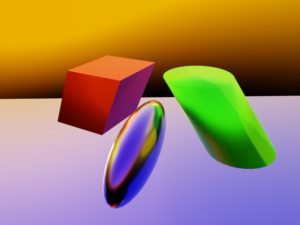We get the impression that some simple geometric shapes like a spat or an ellipsoid could be the direct result of one or more shearing operations applied to originally simple objects with multiple symmetries – as cubes and spheres.

# Shear transformations are mathematically done by unipotent upper triangular matrices

Let us return to a more mathematical description of a shear operation. As any other linear operation applied to vectors of the ℝn we can represent a shear operation by a matrix. We just have to express the linear coupling between two vector components by a suitable arrangement of our shear-constants as matrix-elements. By experimenting a bit we find that a square matrix which only has non-zero elements on its diagonal and in its upper right triangular part does the job. We speak of an “upper triangular matrix“:

$\pmb{\operatorname{M}} \, = \, \begin{pmatrix} m_{11} & m_{12} &\cdots & m_{1n} \\ 0 & m_{22} &\cdots & m_{2n} \\ \vdots &\vdots &\ddots &\vdots \\ 0 & 0 &\cdots & m_{33} \end{pmatrix}$

That such a matrix mixes vector components in the intended way follows directly from the definition of standard matrix multiplications. (Note: In Python/Numpy we talk about “matmul”-operations.)

Note that not all elements in the upper triangular part (aside the diagonal) are required to be different from zero. But at least one should be ≠ 0.

## Not a full and neither a symmetric matrix

Now we come back to a point introduced already above: In principle we could allow for a coupling of two selected vector components in both logically possible ways. E.g., when we have an impact of the z- onto the x-component, why not also allow for an analogous impact of x- onto z at the same time? Maybe with a different linear factor?

Well, we then would get a full linear operation with possibly all n**2 elements having different values or we would get a symmetric matrices. A full generalization is not our objective. We want a special sub-case of a linear transformation. But why not allow for a symmetric matrix?

The reason for this will become clear when we discuss the decomposition and factorization of shear matrices in future posts. For the time being the following hint may help: The complexity of a linear operation can be measured by the minimum number of elementary operations required in a well chosen ECS. Can we find a coordinate system where the transformation reduces to just one elementary operation (translation, scaling, reflection, rotation)?

For a shearing transformation this would have to be a scaling operation as this kind of operation can at least potentially break rotational and planar symmetries.

Now we remember a result of linear algebra: Any symmetric matrix corresponds to an operation for wich we always can find a rotated ECS (at the center of an originally highly symmetric figure) in which the transformation of the figure reduces to a pure scaling operation.

For a shear operation we even want to exclude this kind of reduction: We shall not be able to find a ECS in which the operation just becomes a scaling. (A shearing shall in any coordinate system at least require a scaling and a rotation.) How can we achieve this? Well, we must not even allow for a symmetric matrix. For bodies which show original symmetries with respect to planes through its center this means that a shear operation destroys at least some of its planar symmetries whatever coordinate system we choose. Despite the fact that a shear operation can be inverted (see below)!

So, our elimination of some rotational and at least one planar symmetry in an originally highly symmetric body is reflected by the triangular form of the matrix. We avoid a 2-fold coupling between two selected coordinates at the same time. So when we have a matrix element mi,j, with j > i, we always set the corresponding element to zero: mj,i = 0.

This restricts the distortions we are going to get. Some surface elements are going to remain congruent and some point symmetries are kept up.

## Why did we not use a lower triangular matrix?

Well, this is just a convention. Actually, the transposed matrix of an upper triangular one would also do the job – so we could also take matrices with non-zero elements only in the lower left triangle. But, for convenience, we will stick to matrices with non-zero elements in the upper right triangular part.

## What about the diagonal elements of a shear matrix?

First of all note that not all elements on the diagonal of the matrix Msh should become zero – because then we would eliminate our original figure totally. But for a shear operation we do not want any of the vector components to disappear! In addition: As non-zero elements in the diagonal would reflect a simple scaling we do request that all of the diagonal matrix elements should be equal to 1 for a pure shear operation:

$\pmb{\operatorname{M}}_{sh} \, = \, \begin{pmatrix} 1 & m_{12} &\cdots & m_{1n}(\ne0) \\ 0 & 1 &\cdots & m_{2n} \\ \vdots &\vdots &\ddots &\vdots \\ 0 & 0 &\cdots & 1 \end{pmatrix}$

With at least some of the mi,j(>i) ≠ 0. We then speak of a unipotent or unitriangular matrix.

An example for 4 dimensions would be:

$\pmb{\operatorname{M}}_{sh}^{4D} \: = \: \begin{pmatrix} 1.0 & 0 & 1.5 & 0.8 \\ 0 & 1.0 & 0.2 & 0 \\ 0 & 0 & 1.0 & 2.0 \\ 0 & 0 & 0 & 1.0 \end{pmatrix}$

As the diagonal is fixed by elements mi,i(>i) = 1, we have reduced the number of free and independent (off-diagonal) parameters Nindsh(>i) of a pure shear matrix Msh from n**2 to:

$N^{ind}_{sh}(\pmb{\operatorname{M}}_{sh}) :\: = \: n\,*\, (n\,-\,1)/2$

So, in 2 dimensions instead of 4 parameters we set the diagonal elements to 1 and choose just 1 free off-diagonal parameter. In 3 dimensions we only have to set 3 instead of 6 off-diagonal parameters.

# Basic properties of a shear matrix

A quick calculation shows: The determinant of a shear matrix is directly given by the product of its diagonal elements, and thus we have:

$\operatorname{det} \left(\pmb{\operatorname{M}}_{sh}\right) : = \: 1$

Therefore, a shear matrix is always invertible. This means its effects can be reverted – also in geometrical terms. The matrix also has full rank r = n.

The eigenspace and the eigenvalue(s) of a shear matrix Msh are also interesting. We can use a property of triangular matrices to get the eigenvalue(s):

The diagonal elements of a triangular matrix are its eigenvalues.

This in turn can be understood from analyzing the characteristic polynomial det(M – λI) = 0.

Thus: The one and only eigenvalue ev of Msh is 1.

$\pmb{ev}_{\operatorname{M}_{sh}} \, = \, 1$

The eigenvalues algebraic multiplicity in the characteristic polynom is n This does not yet tell us much about the geometrical multiplicity, i.e. the dimension of the eigenspace.

But all the n eigenvalues share the same eigenspace ES. ES is the kernel of the matrix (A – λI). Therefore, we can derive the eigenspace’s dimension by the dimension formula for this particular matri:

$\operatorname{dim}_{ES} \, = \, n \,- \, \operatorname{rank}\left(\pmb{\operatorname{M}}_{sh} \, – \, \lambda*\pmb{\operatorname{I}} \right) \,=\, n \, – \, (n\,-\,1) \,=\, 1$

as the rank of the combined matrix on the right side is (n – 1).

You also see this understand this by looking at the 4-dim example matrix given above:
When you write down the equations you will find that only 1 variable can be chosen freely. So the kernel of the matrix (A – λI) is only 1-dimensional. Logically and geometrically, this is clear as any other coordinate value (≠ 0) beyond the first one mixes in contributions.

It is easy to prove that the eigenspace ES can be based upon the eigenvector e1.

$\pmb{e}_1 \, = \, \left( \, 1,\, 0,\, \cdots,\,0\right)^T, \: \\ \mbox{with}\, (n\,-\, 1) \, \mbox{ zero components}$

# A shear matrix cannot be diagonalized

Can Msh be diagonalized? Answer: No! From linear algebra we know that this would require n linearly independent eigenvectors. But we have just one! So:

$\pmb{\operatorname{M}}_{sh} : \neq \: \pmb{\operatorname{Q}} \circ \pmb{\operatorname{D}}_{sh} \circ \pmb{\operatorname{Q}^T}$

Think a bit about the consequences:
An operation like that on the right side of the un-equation above would correspond to the representation of our shear matrix in a different (rotated) coordinate system. A diagonalization corresponds to an eigendecomposition; in geometrical terms a diagonal matrix represents a pure scaling operation. But, obviously, such a description of our shear operation in some (cleverly) selected ECS is not possible:

We cannot find a (translated and rotated) ECS in which a shear operation reduces to a scaling transformation. In any chosen (rotated) ECS there is always an additional second rotation involved which we cannot simply invert or get rid off again!

We shall see this in more detail when we discuss decompositions. We simply cannot get rid of the fundamental asymmetry we have build into Msh by not allowing non-zero elements in the lower triangular part of the matrix! No change to a whatever selected coordinate system will help.

So, a shear operation really is a special symmetry breaking operation which cannot be reduced to just one elementary operation. In any ECS it requires at least a rotation and a scaling!

Summary:

A pure shear operation is introduced by some off-diagonal and non-zero element in the upper right triangular part of a unipotent matrix. All off-diagonal elements in the lower left triangular part are set to zero.

# More illustrations from Blender

First some images for a cuboid that was more extremely deformed by shearing in x- and y-direction than the first one: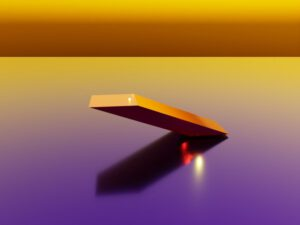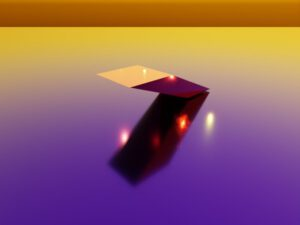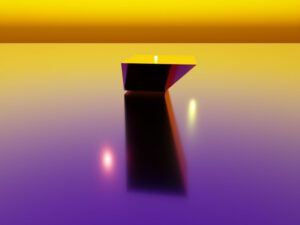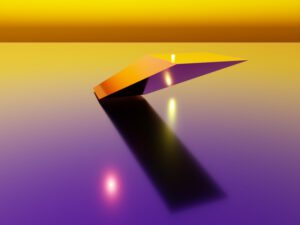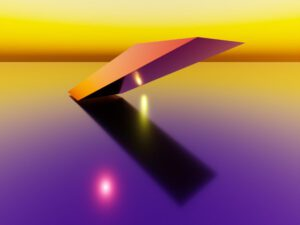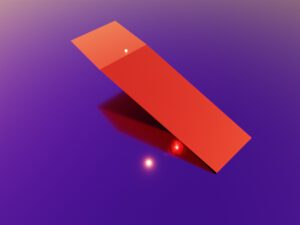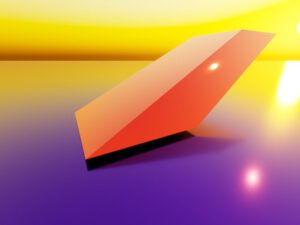Now we come to an interesting example: We apply shearing in x- and y-direction to a sphere. We get something whose shape looks much more regular than that of a cuboid. We pick a unit sphere and apply to shearing operations in x- and y-direction: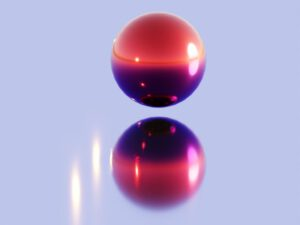The first image shows the original sphere from some point of view. The second image was taken from the same perspective, but now after the shearing. The resulting figure looks very regular – and it seems to have plane symmetries.

Another example with a bit of different lighting gives us:Actually, we get an ellipsoid. We clearly can identify the two points which have a maximum absolute z-values whose tangent planes did not change tier orientation.

Ellipsoids (in 3D) are figures with 3 main axes and planar symmetries. The original fully rotational symmetry (first image of the series above) is obviously broken. However, we seem to have saved at least some planar symmetries. Hmmm …. Just when we thought we had understood that a shearing operation breaks original planar symmetries we have in our new example case kept some.

Why is this? What is so special about the shearing of spheres? Keep this question in mind.

# Conclusion

Shearing transformations are a special class of linear and thus transformations. Shearing obviously can break some plane symmetries of originally highly symmetric bodies – as cubes. In contrast to other elementary affine operations no coordinate system can be found where the number of operations to reproduce the effects of a shearing can be reduced to one. In particular it is not possible to find an Euclidean coordinate system where it is just reduced to a (symmetry breaking) scaling with different factors in different coordinate directions.

Blender offers a simple graphical method to apply shearing to simple geometrical bodies. This allowed us to get an impression of the effects on cubes and spheres. Something that was a bit astonishing was the shearing effect on a unit spheres. It just created ellipsoids – again highly symmetric bodies WITH planar symmetries.

In the next post of this series
Fun with shear operations and SVD – II – Shearing of rectangles and cubes with Python and Matplotlib
we will look closer at shearing operation with the help of Python and matplotlib.

# Flatpak and Blender after system updates – update the flatpak Nvidia packet to match your drivers

Some days ago I wanted to perform some Blender experiments on my laptop. Before I had upgraded most of the installed Leap 15.3 packages installed. Afterwards I thought this was a good opportunity to bring my flatpak based installation of Blender up to date, too.

A flatpak installation of Blender had been necessary since I had changed the laptop’s OS to Opensuse Leap 15.3. Reason: Opensuse did and does not offer any current version of Blender in its official repositories for Leap 15.3 (which in itself is a shame). (I have not yet checked whether the situation has changed with Leap 15.4.)

Blender’s present version available for flatpak is 3.3.1. I had Blender version 3.1 installed. So, I upgraded to version 3.3.1. However, this upgrade step for Blender alone did not work. (In contrast to a snap upgrade).

# The problem

The sequence of commands I used to perform the update was:

flatpak remote-add --if-not-exists flathub https://flathub.org/repo/flathub.flatpakrepo
flatpak update org.blender.Blender
flatpak list


The second command brought Blender V3.3.1 to my disk. However, when I tried to start my new Blender with

flatpak run org.blender.Blender &


the system complained about a not working GLX and GL installation.

But, actually my KDE desktop was running on the laptop’s Nvidia card. The laptop has an Optimus configuration. I use Opensuse Prime to switch between the Intel i915 driver for the graphics card integrated in the processor and the Nvidia driver for the dedicated graphics card. And Nvidia was running definitively.

# The cause of the failure and its solution

Flatpak requires the right interface for the Nvidia card AND the presently active GLX-environment to start OpenGL applications.

A “flatpak list” showed me that I had an “app” “nvidia-470-141-01” running.

Name                    Application ID                                   Version    Branch   Installation
Blender                 org.blender.Blender                              3.3.1      stable   system
Codecs                  org.blender.Blender.Codecs                                  stable   system
Freedesktop Platform    org.freedesktop.Platform                         21.08.16   21.08    system
Mesa                    org.freedesktop.Platform.GL.default              21.3.9     21.08    system
nvidia-470-141-01       org.freedesktop.Platform.GL.nvidia-470-141-01               1.4      system
Intel                   org.freedesktop.Platform.VAAPI.Intel                        21.08    system
ffmpeg-full             org.freedesktop.Platform.ffmpeg-full                        21.08    system
openh264                org.freedesktop.Platform.openh264                2.1.0      2.0      system


A quick view to the nvidia-settings and YaST showed me, however, that the drivers and other components installed on Leap 15.3 were of version “nvidia-470-141-03”.

Then I tried

mytux:~ # flatpak install flathub org.freedesktop.Platform.GL.nvidia-470-141
Looking for matches…
Similar refs found for ‘org.freedesktop.Platform.GL.nvidia-470-141’ in remote ‘flathub’ (system):

1) runtime/org.freedesktop.Platform.GL.nvidia-470-94/x86_64/1.4
2) runtime/org.freedesktop.Platform.GL.nvidia-470-141-03/x86_64/1.4
3) runtime/org.freedesktop.Platform.GL.nvidia-470-141-10/x86_64/1.4
4) runtime/org.freedesktop.Platform.GL.nvidia-470-74/x86_64/1.4
5) runtime/org.freedesktop.Platform.GL.nvidia-430-14/x86_64/1.4
6) runtime/org.freedesktop.Platform.GL.nvidia-390-141/x86_64/1.4

Which do you want to use (0 to abort)? [0-6]: 2


This command offered me a list to select the required subversion from. In my case option 2 was the appropriate one.

And indeed after the installation I could start my new Blender version again.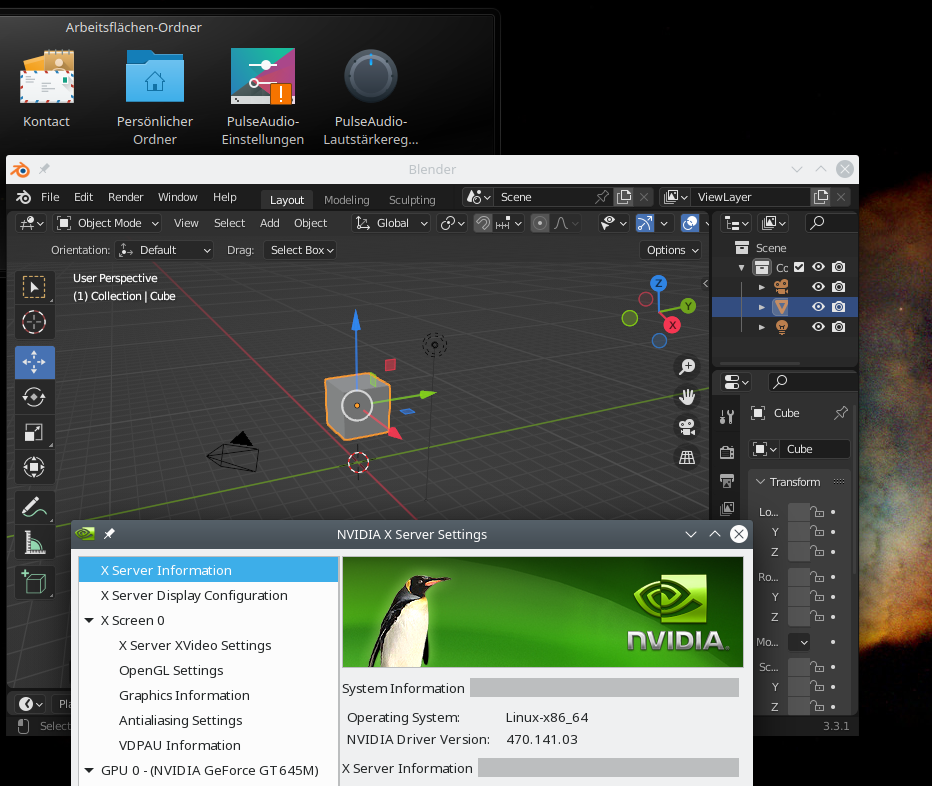By the way: Flatpak allows for multiple versions to be installed at the same time. Like:

Name                 Application ID                                Version  Branch Installation
Blender              org.blender.Blender                           3.3.1    stable system
Codecs               org.blender.Blender.Codecs                             stable system
Freedesktop Platform org.freedesktop.Platform                      21.08.16 21.08  system
Mesa                 org.freedesktop.Platform.GL.default           21.3.9   21.08  system
nvidia-470-141-03    org.freedesktop.Platform.GL.nvidia-470-141-03          1.4    system
nvidia-470-141-10    org.freedesktop.Platform.GL.nvidia-470-141-10          1.4    system
Intel                org.freedesktop.Platform.VAAPI.Intel                   21.08  system
ffmpeg-full          org.freedesktop.Platform.ffmpeg-full                   21.08  system
openh264             org.freedesktop.Platform.openh264             2.1.0    2.0    system


# Conclusion

Your flatpak installation must provide a version of the ‘org.freedesktop.Platform.GL.nvidia-xxx-nnn-mm’ packet which matches the present Nvidia driver installation on your Linux operative system.
Do not forget to upgrade flatpak packets for NVidia after having upgraded Nvidia drivers on your Linux OS!